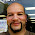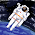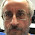## Friday, May 30, 2014

### HP Prime: Ways to use the INPUT command (Version 6030)

Note: This is for HP Prime Software Version 6030 (2014 3 31)

The INPUT Command

On the HP Prime, the INPUT command can include various types of input forms: numeric fields, choose boxes, and check boxes. You can also restrict which types of input are accepted. This blog entry will cover some of the basic forms of INPUT.

Basic Form

INPUT(var, title, label);

var = can be one or a list
title = optional string
label = optional string, number of labels are equal to number of var

The example programs presented (except for IN4) win use one variable.

Here is an example of the basic format. Keep in mind, the title and label strings are optional.

EXPORT IN1( )
BEGIN
// Basic
INPUT(X, "Enter X");
RETURN √(1+X^2);
END;

Specifying Types

INPUT( {var, [types allowed]}, title, label);

Type numbers are surrounded in square brackets.

Common Types:
-1 All
0 real numbers
1 integers
2 strings
3 complex numbers
4 matrices
6 lists
8 functions
9 numbers with units
14.? CAS objects

IN2 and IN3 are programs that ask for a specific type. IN2 asks for a matrix (type 4) and IN3 asks for a list (type 6).

EXPORT IN2( )
BEGIN
INPUT({{M0,}}, "Matrix");
RETURN M0*TRN(M0);
END;

EXPORT IN3( )
BEGIN
INPUT({{L0, }}, "List");
PRINT( );
PRINT("Σx ="+STRING(ΣLIST(L0)));
PRINT("mean = "+STRING(approx(mean(L0))));
PRINT("std dev ="+STRING(approx(steddevp(L0))));
END;

Checkboxes

INPUT({{var, n}}, title, label);

n groups the current and next n-1 checkboxes. If you want a checkbox next to each variable, let n = 1. A variable that is checked has a value of 1. Otherwise, the value is 0. Each variable and it's corresponding n are surrounded in curly brackets.

EXPORT IN4( )
BEGIN
// Checkboxes
INPUT( {{A,1}, {B,1}, {C,1}}, "Turn On Variables");
PRINT( );
PRINT("Number of Checks: "+STRING(A+B+C));
END;

If you want to use more than one input form, I advise to keep INPUT types separate.

EXPORT IN5( )
BEGIN
// Checkbox Modes
INPUT( {{M,1}}, "Check for Degrees");
HAngle:=M;
// Input Data
INPUT(A, "Angle");
PRINT( );
PRINT("SIN(2A)² =");
PRINT(SIN(2*A)²);
END;

Choose Boxes

This INPUT form will create a choose box (drop down list).

INPUT( {{ var, {choice0, choice1, choice2...} }}, title, label)

IF var == 0 THEN choice0 instructions...(END;)
ELSE (if there are only two choices) or
IF var ==1 THEN choice1 instructions... END; and so on...

EXPORT IN6( )
BEGIN
INPUT( {{C, {"SI","US"} }}, "Units of g");
IF C==0 THEN
RETURN "g = 9.80665 m/s^2"; KILL;
ELSE
RETURN "g = 32.17404 ft/s^2"; END;
END;

That is some of the ways we can use the INPUT command on the HP Prime. To check out all of the ways INPUT command, including putting the prompts in specific places, check out the help screen for INPUT.

Eddie

This blog is property of Edward Shore. 2014

1.Hello!
In my case ''advanced'' function of INPUT command doesn't work. No checkboxes, no choose boxes, no specifying type. I get the message ''Error: Invalid input''.

Have you idea what's wrong with my HP Prime?

The classic way of INPUT command (INPUT(var, title, label);)works OK

Thank you very much in advance!

BTW: Very good and useful blog!

1.INPUT is a very funny command. Unfortunately I ended up using the most basic form of INPUT (asking for a real value to a global or local variable). Hopefully when the software of the HP Prime gets updated, INPUT will be cooperate better.

Eddie

2.hello,
Post a bug in the program line

INPUT({{M0,}}, "Matrix");

Error:Invalid input

what could be the problem?

3.Reaction to Andrey Kumko

Maybe you entered MO instead of M0, with O a letter instead of a zero.
It should be a zero because M0 is already a variable and MO has not yet been declared as a variable.

When you still want to use MO you have to declare it as a variable before, eg by: LOCAL MO;

4.Reaction to Kramp

Are you sure you used double curly brackets?

5.You can now add help text to the INPUT command. See below. In the case of multiple inputs, you can use a list of help messages, one for each variable input.

EXPORT IN1( )
BEGIN
// Basic
INPUT(X, "Enter X","X","Type a value for X PLEASE!");
RETURN √(1+X^2);
END;

2.This comment has been removed by the author.

3.Hi Edward,

Thank you for this useful information concerning the INPUT command.
I read the online user manual and this says that in order to specify the allowed variable types you must enter a parameter called [allowed_types_matrix].

I was wondering what this was and tried all kind of things, without succes.

Your Blog gave the answer: to specify an allowed type you have to enter a number between square brackets, eg for matrices the number 4.

This solved the problem for me but I am wondering how you got to know this?

I cannot find it anywhere in the help files or am I missing something?

4.How to set the input to enter an angle value in HMS that the press recognizes the value in HMS.
Thank you

5.It is easy.
When you want to enter 5 degrees, 13 minutes, 34 seconds, enter:
5 (shift E) 13 (shift E) 34 (shift E)

The result is shown in the format you wanted.
You can also switch between HMS view and normal view by repeatedly pressing (shift E).

Read the online user manual! A must for this calculator.

6.Another exemple with 2 complex numbers:

EXPORT Specifying_Types_complex()
BEGIN
LOCAL AnyVar,AnyVar2;
INPUT({{AnyVar,},{AnyVar2,}}, "Complex");
RETURN AnyVar*AnyVar2;
END;

7.Para que se utuilizan los types  y type [14?.].

Estoy intentando hacer un programa en el que la entrada a una variable (LOCAL long; //longitud ) sea una variable algebraica: l. El caso es que si le pongo [-1], si escribo return long; me devuelve "l", identificándolo como una cadena. Si en una linea más abajo pongo A:=long*long; me da error en lugar de devolver l^2 . Llevo toda la semana con esto, y sé que poder se puede, ya que en modo CAS si usted escribe l*l le da L^2. Es más, si yo programo en modo CAS de la forma:
#cas
ejempli():=
BEGIN
local l,Long;
Long:=l; //como sustituir esto por un input?
A:=long*long;
return A;
END;
#end
el programa me retorna l^2. Pero en el momento en el que cambio la línea comentada por: input({long,[-1]}); si le pongo algo que no sea un número no lo interpreta como un valor algebraico :(

No se si me he explicado bien :( Gracias por ayudar

### TI-84 Plus CE: Simple Elliptic Curves Determined by Two Points

TI-84 Plus CE:  Simple Elliptic Curves Determined by Two Points Introduction The program ECURVE determines the coefficients of a simple...